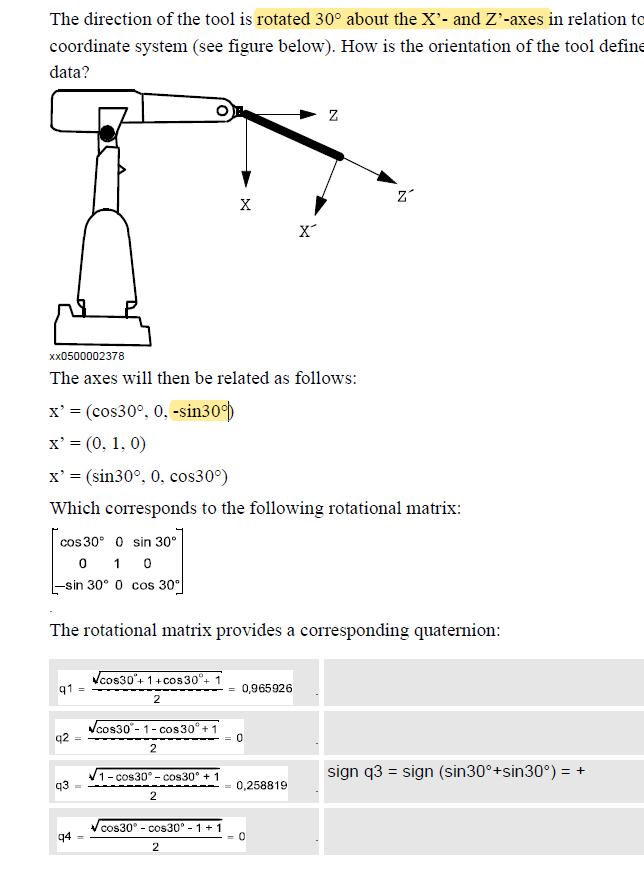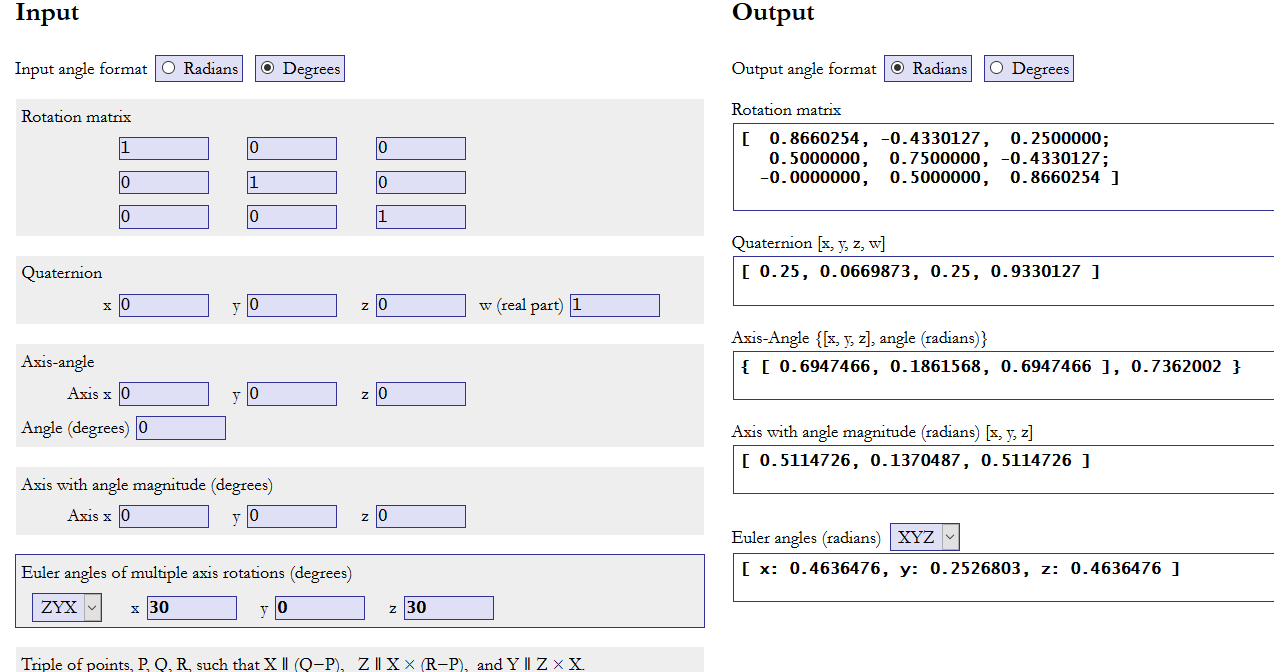Manual quaternion example gives me a different result? What am I missing?

Just in general:
If i try run the example below i have a different result than the manual:
Mine is 1.93185,  0, 0.517638, 0.
Am i making a mistake? Is the example correct? Any input appreciated

double q1 = sqrt(cos(0.523599)+ 1 + cos(0.523599) +1);
double q2 = sqrt(cos(0.523599) -1 - cos(0.523599) + 1);
double q3 = sqrt(1 - cos(0.523599) - cos(0.523599) + 1);
double q4 = sqrt(cos(0.523599) - cos(0.523599) -1 + 1);• Closer but that would be [ 0.2588223, 0, 0.9659249, 0 ] in abb notation?
Abb notation is x, y, z, w?The result in the example looks correct (middle  not rotated) unit but how?
const robtarget test2_1_l0:=[[-0.52, -30.00, 10.13], [0.9659249, 0, 0.2588223, 0],[-1,0,-1,0], [9E+09,9E+09,9E+09,9E+09,9E+09,9E+09]];
const robtarget test2_2_l0:=[[4.18, -29.71, 10.26], [0, 0.2588223, 0, 0.9659249],[-1,0,-1,0], [9E+09,9E+09,9E+09,9E+09,9E+09,9E+09]]The doc doesnt exactly explain the notation?
Components
The data type orient has the following components:
q1
Data type: num
Quaternion 1.
q2
Data type: num
Quaternion 2.
q3
Data type: num
Quaternion 3.
q4
Data type: num
Quaternion 4.

Post edited by k_schmid on
• Can we just confirm this is  a correct example and the notation?
Will robotstudio deal with scientific notation like 5.68811e-14? I dont think it did in my quick test.

The calculator show above is this
https://www.andre-gaschler.com/rotationconverter/

Post edited by k_schmid on
• I think the Euler angles are in the order Z,Y,X.
As there is a RAPID function EulerZYX which obtains the rotation angles from the quaternions and OrientZYZ that build the quaternions.
• So we think the result is correct?  Or that quaternions are q= [w,Z,Y,X]?Or both?
I am using libigl, a  proven library and have trouble to make any sense of the results in robotstudio

• Here is the result with EulerZYX• With const robtarget test2_1_l0:=[[-0.52, -30.00, 10.13], [0.965926, 0, 0.258819, 0],[-1,0,-1,0], [9E+09,9E+09,9E+09,9E+09,9E+09,9E+09]];
only y has an angle• I am not any good at the math involved in this....
So I went online found a converter which used ZYX for the Euler input and plugged in 30,0,30 - got a completely different result to the manual extract!
So had a good look at the diagram of the robot diagram in your extract from the manual it looks like a 30° rotation round the Y axis.
You plug those numbers into the converter  ( z= 0, y=30, x=0) you get the result shown in the manual.

What was the manual you got the extract from and I will raise it as a correction?
• Thanks so much, that matches robotstudios result. The example this is page 1152
3 Data types
3.37. orient - Orientation
RobotWare - OS
in
ABB Robotics
Technical reference manual
RAPID Instructions, Functions and Data types

I assume there isnt anything else available? No i know howit doesnt work mainly

Post edited by k_schmid on
• Can somebody confirm  robotstudio has a right-handed coordinate system?

• rotations are in world space? parent space?
so my coordinate system is z down, right handed , ZYX order...